# PSEB 7th Class Maths Solutions Chapter 10 Practical Geometry Ex 10.5

Punjab State Board PSEB 7th Class Maths Book Solutions Chapter 10 Practical Geometry Ex 10.5 Textbook Exercise Questions and Answers.

## PSEB Solutions for Class 7 Maths Chapter 10 Practical Geometry Ex 10.5

1. Construct a right angled triangle ABC with ∠C = 90°, AB = 5 cm and BC = 3 cm.
Solution:
Given : Two sides of ΔABC as
AB = 5 cm,
BC = 3 cm
and ∠C = 90°.
To construct : A triangle with these two sides and one right angle.
Steps of Construction :
Step 1. We first draw a rough sketch of the triangle ABC and indicate the measure of these two sides and mark the right angle.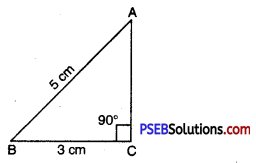Step 2. Draw BC of length 3 cm.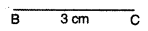Step 3. At C, draw CX ⊥ BC. (A should be somewhere on this perpendicular).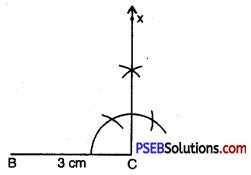Step 4. With B as centre, draw an arc of radius 5 cm. (A must be on this arc since it is at a distance of 5 cm from B).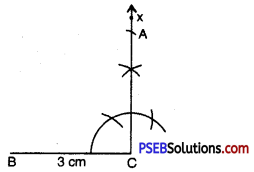Step 5. A has to be on the perpendicular line CX as well as on the arc drawn with centre C.
∴ A is the meeting point of these two.
ΔABC is now obtained.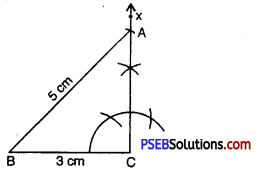2. Construct an isosceles right angled triangle DEF where ∠E = 90° and EF = 6 cm.
Solution:
Given : An isoscele right angled ΔDEF where ∠E = 90° and EF = 6 cm.
To Construct: A right angled triangle with one side.
Steps of Construction:
Steps 1. Draw a rough sketch of given measures.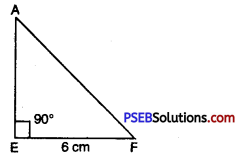Step 2. Draw a line segment EF = 6 cm.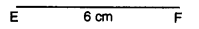Step 3. With the help of compass taking E as centre, draw a ray EX making an angle of 90° with EF.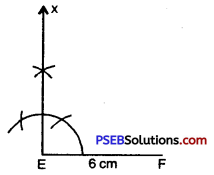Step 4. With E as centre and radius 6 cm (= DE) draw an arc intersecting EX at D.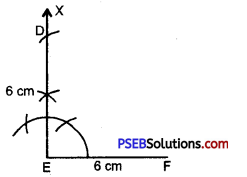Step 5. Join D and F. Therefore ΔDEF is required isosceles right triangle.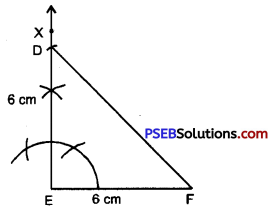3. Construct a right-angled triangle PQR in which :
∠Q= 90°, PQ = 3.6 cm and PR = 8.5 cm
Solution:
Given : Right triangle be PQR; right-angled at Q
i. e. ∠Q = 90°
and PQ = 3.6 cm,
PR = 8.5 cm
To construct : A triangle with these two sides and one right angle.
Steps of Construction :
Step 1. We first draw a rough sketch of the triangle PQR and indicate the measure of these two sides and mark the right angle.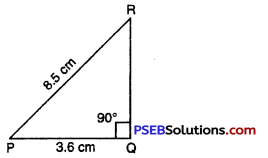Step 2. Draw PQ of length 3.6 cm.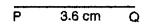Step 3. At Q, draw QX ⊥ PQ.
(R should be somewhere on this perpendicular).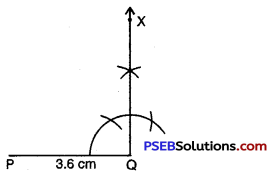Step 4. With P as centre, draw arc of radius
(R must be on this arc, since it is at a distance of 8.5 cm from P).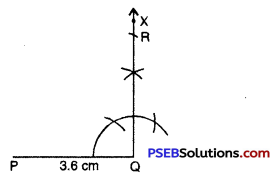Step 5. R has to be on the perpendicular line QX as well as on the arc drawn with centre P.
∴ R is the meeting point of these two.
ΔPQR is now obtained.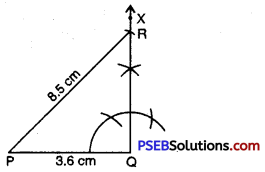4. Question (i).
Which of the following is a pythagorian triplet ?
(a) 1, 2, 3
(b) 2, 3, 4
(c) 4, 5, 6
(d) 12, 13, 5